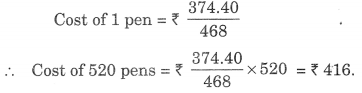# Ratio and Proportion Class 6 Extra Questions Maths Chapter 12

ncert textbook

## Ratio and Proportion Class 6 Extra Questions Maths Chapter 12

Extra Questions for Class 6 Maths Chapter 12 Ratio and Proportion

### Ratio and Proportion Class 6 Extra Questions Very Short Answer Type

Question 1.
Find the ratio of 75 cm to 1.5 m.
Solution:
The given numbers are not in the same units. So, converting them into same units.
1.5 m = 1.5 x 100 cm = 150 cm
[∵ 1 m = 100 cm]
∴ The required ratio is 75 cm : 150 cm.∴ Required ratio = 1 : 2

Question 2.
Give two equivalent ratios of 3 : 5.
Solution: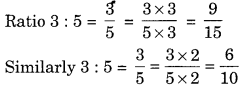Thus, 9 : 15 and 6 : 10 are the two equivalent ratios of 3 : 5.

Question 3.
Fill in the blank box.Solution:Question 4.
Check whether the given ratios are equivalent or not.$\frac { 2 }{ 7 }$,$\frac { 6 }{ 21 }$
Solution: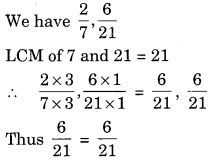∴ They are equivalent ratios.

Question 5.
Divide 60 in the ratio of 2 : 3.
Solution:
Sum = 2 + 3 = 5
∴ First part =$\frac { 2 }{ 5 }$x 60 =24 5
∴ Second part =$\frac { 3 }{ 5 }$x 60 =36 5
Thus, the required two parts = 24 and 36.

Question 6.
Find the ratio of the following:
(a) 56 to 63.
(b) 55 to 120.
Solution:Question 7.
Ramesh deposited ₹ 2050 in a bank and in the month of January he withdrew ₹ 410 from his account on the last date of the month. Find the ratio of
(a) Money withdrawn to the total money deposited.
(b) Money withdrawn to the remaining amount in the bank.
Solution:
Total money deposited = ₹ 2050
Amount of money withdrawn = ₹ 410
Amount of money left in the bank = ₹ 2050 – ₹ 410 = ₹ 1640
(a) Ratio of money withdrawn to the total money deposited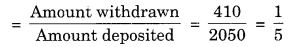∴ Required ratio = 1 : 5
(b) Ratio of money withdrawn to the money left in the bank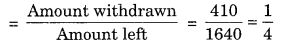∴ Required ratio = 1 : 4

Question 8.
There are 180 students in a class. Number of girls are 75. Find the ratio of the girls to the number of boys.
Solution:
Total number of students = 180
Number of girls = 75
Number of boys = 180 – 75 = 105
∴ Ratio of number of girls to the number of boysRequired ratio = 5 : 7

### Ratio and Proportion Class 6 Extra Questions Short Answer Type

Question 9.
Green paint is made by mixing blue, yellow and white paints in the ratio 2 : 7 : 1. How much blue paint is needed to make 64 litres of green paint?
Solution:
Here, sum of ratios = 2 + 7 + 1 = 10
∴ Total quantity of green paint = 64 litres
Quantity of blue paint =$\frac { 2 }{ 10 }$x 64 = 12.8 litres
Therefore, the required blue paint = 12.8 litres.

Question 10.
From the figure, find the ratio of
(a) The number of squares to the number of triangles.
(b) The number of circles to the number of rectangles.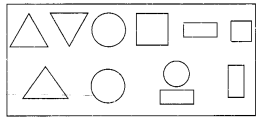Solution:
(a) Number of squares = 2
Number of triangles = 3 2
∴ Ratio =$\frac { 2 }{ 3 }$or 2 : 3

(b) Number of circles = 3
Number of rectangles = 3
∴ Ratio =$\frac { 3 }{ 3 }$or 1 : 1

Question 11.
In each of the following figures, find the ratio of the shaded region to the unshaded region.Solution:
(a) Number of shaded parts = 4
Number of unshaded parts = 12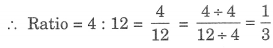Required ratio = 1 : 3

(b) Number of shaded parts = 2
Number of unshaded parts = 4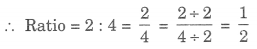Required ratio = 1 : 2

Question 12.
Are 20, 25, 12, 15 in proportion?
Solution:
We have 20, 25, 12, 15
Product of extremes = 20 x 15 = 300
Product of middles = 25 x 12 = 300
Since both the products are same.
∴ The four numbers 20, 25, 12, 15 are in proportion.

Question 13.
The first, second and fourth terms in a proportion are 32, 112, 217 respectively. Find the third term.
Solution:
Let the third term be x.
∴ 32, 112, x and 217 are in proportion.
∴ 32 : 112 :: x : 217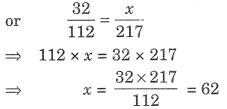Thus, the third term = 62.

Question 14.
Find the value of x, if
(а) 8, x, x, 50 are in proportion.
(b) 36, 90, 90, x are in proportion.
Solution:
(a) Since 8, x, x, 50, are in proportion.
∴ x × x = 8 × 50
⇒ x2 = 400
∴ x = 20

(b) Since 36, 90, 90, x are in proportion.
∴ 36 × x = 90 × 90
⇒ x =$\frac { 90\times 90 }{ 36 }$= 225
∴ x = 225

Question 15.
The cost of 10 tables is ₹ 7500. Find the number of tables that can be purchased with ₹ 9000.
Solution:
Number of tables purchased in ₹ 7500 = 10
Number of tables purchased in ₹ 1 =$\frac { 10 }{ 7500 }$
∴ Number of tables purchased in ₹ 9000
=$\frac { 10\times 9000 }{ 7500 }$= 12

Question 16.
39 packets of 12 pens each costs ₹ 374.40. Find the cost of 52 packets of 10 pens each.
Solution:
Number of pens in 1 packet = 12
Number of pens in 39 packets = 12 x 39 = 468
Number of pens in 1 packet = 10
Number of pens in 52 packets = 10 x 52 = 520
Now cost of 468 pen = ₹ 374.40